## Thursday, 7 August 2014

### chapter-10 - Worked Out Examples – 3

 Example: 5
A fair coin is tossed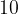$10$ times. Find the probability of obtaining
 (a) exactly$6$ Heads (b) at most$6$ Heads (c) at least$6$ Heads
 Solution: 5-(a)
Consider any arbitrary sequence of$10$ tosses that contains exactly$6$ Heads. For example, consider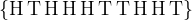${\rm{\{ H\, T\, H\, H\, H \,T \,T \,H \,H\, T\} }}$
Any such sequence has a probability of occurrence equal to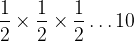$\dfrac{1}{2} \times \dfrac{1}{2} \times \dfrac{1}{2}\ldots 10$ times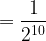$= \dfrac{1}{{{2^{10}}}}$ Thus, what we need to do is count the number of sequences with exactly$6$ Heads. The required probability will then be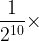$\dfrac{1}{{{2^{10}}}} \times$ (No. of sequences).
Counting such sequences is a simple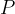$P$&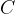$C$ problem, and those familiar with that subject will immediately hit upon the answer: there are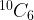${}^{10}{C_6}$ such sequences.
Thus,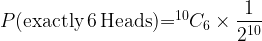$P ({\rm{exactly\,}} 6 \,{\rm{Heads}}){ = ^{10}}{C_6} \times \dfrac{1}{{{2^{10}}}}$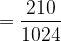$= \dfrac{{210}}{{1024}}$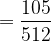$= \dfrac{{105}}{{512}}$
In general, we see that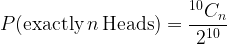$P ({\rm{exactly}} \,n\,{\rm{ Heads}}) = \dfrac{{^{10}{C_n}}}{{{2^{10}}}}$
 Solution: 5-(b)
For this question, we consider all the possible cases and add their respective probabilities like this: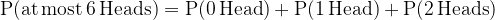${\rm{P(at\, most\, 6 \,Heads) = P(0\, Head)+ P(1\, Head) + P(2\, Heads}})$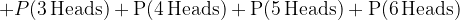$+ P(3 \,{\rm{Heads) + P(4 \,Heads) + P(5\, Heads) + P(6\, Heads)}}$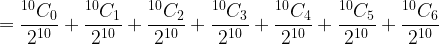$= \dfrac{{^{10}{C_0}}}{{{2^{10}}}} + \dfrac{{^{10}{C_1}}}{{{2^{10}}}} + \dfrac{{^{10}{C_2}}}{{{2^{10}}}} + \dfrac{{^{10}{C_3}}}{{{2^{10}}}} + \dfrac{{^{10}{C_4}}}{{{2^{10}}}} + \dfrac{{^{10}{C_5}}}{{{2^{10}}}} + \dfrac{{^{10}{C_6}}}{{{2^{10}}}}$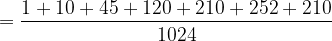$= \dfrac{{1 + 10 + 45 + 120 + 210 + 252 + 210}}{{1024}}$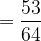$= \dfrac{{53}}{{64}}$
 Solution: 5-(c)
Similarly, we have${\rm{P(at\, least\, 6\, Heads) = P(6 \,Heads) + P(7 \,Heads) + P(8 \,Heads) + P(9 \,Heads) + P(10\, Heads)}}$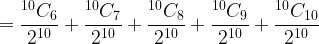$= \dfrac{{^{10}{C_6}}}{{{2^{10}}}} + \dfrac{{^{10}{C_7}}}{{{2^{10}}}} + \dfrac{{^{10}{C_8}}}{{{2^{10}}}} + \dfrac{{^{10}{C_9}}}{{{2^{10}}}} + \dfrac{{^{10}{C_{10}}}}{{{2^{10}}}}$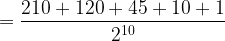$= \dfrac{{210 + 120 + 45 + 10 + 1}}{{{2^{10}}}}$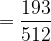$= \dfrac{{193}}{{512}}$
This questions was an example of Binomial Distributions, something we’ll study in more detail later in this chapter.
 Example: 6
 Consider the sequence of numbers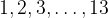$1,2,3,\ldots ,13$. A person chooses three numbers at random from this sequence. Find the probability that the three numbers form an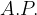$A.P.$
 Solution: 6
Note that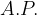$A. P.$s can be formed with varying common differences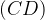$(CD)$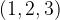$(1, 2, 3)$ and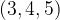$(3, 4, 5)$ are examples of$A. P.$s with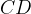$CD$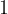$1$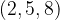$(2, 5, 8 )$ is an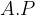$A. P$of$CD$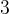$3$. The maximum$CD$ possible is$6$, in the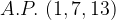$A. P.\,\, (1, 7, 13)$.
Let us count all such$A. P.$s in a table. Verify the column on the right.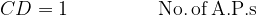$CD=1 \,\,\,\,\,\,\,\,\,\,\,\,\,\,\,\,\,\,\,\,\,\, \,\,\,\,\,{\rm{No.\, of \, A.P.s}}$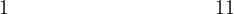$1\,\,\,\,\,\,\,\,\,\,\,\,\,\,\,\,\,\,\,\,\,\,\,\,\,\,\,\,\,\,\,\,\,\,\,\,\,\,\,\,\,\,\,\,\,\,\,\,\,\,\,\,\,\,\,\,\,\,\,\,\,\,11$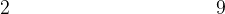$2\,\,\,\,\,\,\,\,\,\,\,\,\,\,\,\,\,\,\,\,\,\,\,\,\,\,\,\,\,\,\,\,\,\,\,\,\,\,\,\,\,\,\,\,\,\,\,\,\,\,\,\,\,\,\,\,\,\,\,\,\,\,9$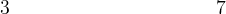$3\,\,\,\,\,\,\,\,\,\,\,\,\,\,\,\,\,\,\,\,\,\,\,\,\,\,\,\,\,\,\,\,\,\,\,\,\,\,\,\,\,\,\,\,\,\,\,\,\,\,\,\,\,\,\,\,\,\,\,\,\,\,7$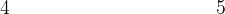$4\,\,\,\,\,\,\,\,\,\,\,\,\,\,\,\,\,\,\,\,\,\,\,\,\,\,\,\,\,\,\,\,\,\,\,\,\,\,\,\,\,\,\,\,\,\,\,\,\,\,\,\,\,\,\,\,\,\,\,\,\,\,5$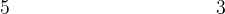$5\,\,\,\,\,\,\,\,\,\,\,\,\,\,\,\,\,\,\,\,\,\,\,\,\,\,\,\,\,\,\,\,\,\,\,\,\,\,\,\,\,\,\,\,\,\,\,\,\,\,\,\,\,\,\,\,\,\,\,\,\,\,3$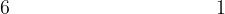$6\,\,\,\,\,\,\,\,\,\,\,\,\,\,\,\,\,\,\,\,\,\,\,\,\,\,\,\,\,\,\,\,\,\,\,\,\,\,\,\,\,\,\,\,\,\,\,\,\,\,\,\,\,\,\,\,\,\,\,\,\,\,1$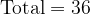${\rm{Total}}=36$
Thus, the total number of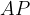$AP$s possible from this set is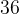$36$. Also, from this set of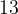$13$ numbers, a selection of$3$ numbers can be made in${}^{13}{C_3} = 286$ways.
Therefore, the probability that three numbers picked at random from this set form an$A.P.$ is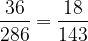$\dfrac{{36}}{{286}} = \dfrac{{18}}{{143}}$.